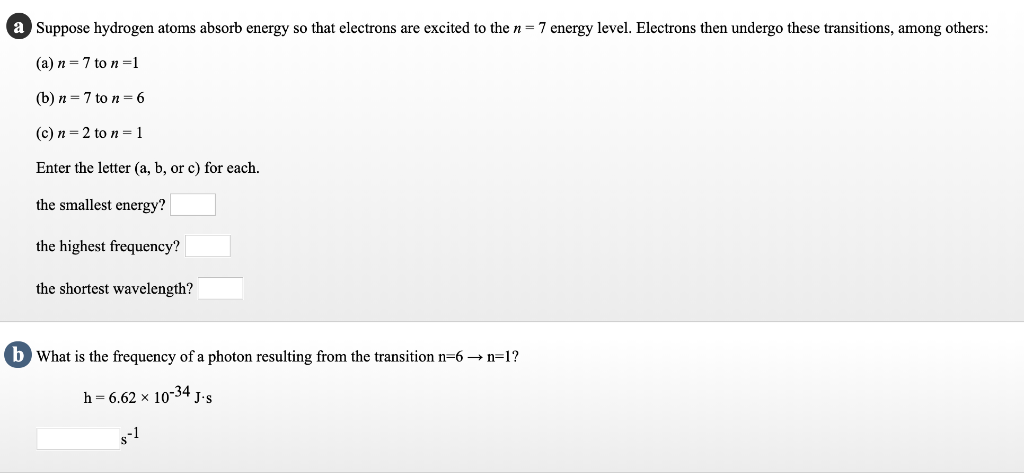Homework Help Question & Answers

# 4 Suppose hydrogen atoms absorb energy so that electrons are excited to the n-7 energy level. Ele...4 Suppose hydrogen atoms absorb energy so that electrons are excited to the n-7 energy level. Electrons then undergo these transitions, among others (a) n 7 to n- (b) n 7 to n-6 (c) n-2 to n-1 Enter the letter (a, b, or c) for each. the smallest energy? the highest frequency? the shortest wavelength? What is the frequency of a photon resulting from the transition n-6 → n-1? h -6.62 x 10-34 J-s

The smallest energy?

The smallest energy Of photon partical when electron jumps in n = 6 from n = 7, because when we move n= 1 from above we see the energy gape between them is Decreasing ,and you also understand from

E is propasanal to 1/(n final )2 - 1/( n in) 2

The highest frequency?

Higher will energy gap higher will frequency

So answer is n = 7 to n = 1

The shortest wavelenght?

There is also higher will energy gap shorteSt the wave lenght, because E = h x frequency = h x c /wavelenght so answer is n= 7 to n = 1

In question B

Frequency = R X C x 1/( n fi) 2 - 1/( n in) 2

Where R = Rydberg constant

C = speed of lightF =##### Add Answer of: 4 Suppose hydrogen atoms absorb energy so that electrons are excited to the n-7 energy level. Ele...
More Homework Help Questions Additional questions in this topic.

• #### quantum level for excited electrons

Need Online Homework Help?

Get FREE EXPERT Answers
WITHIN MINUTES
Related Questions